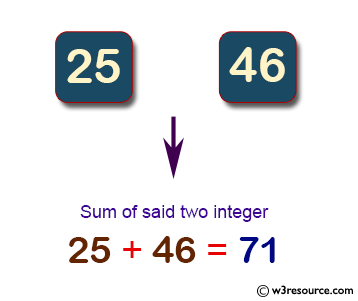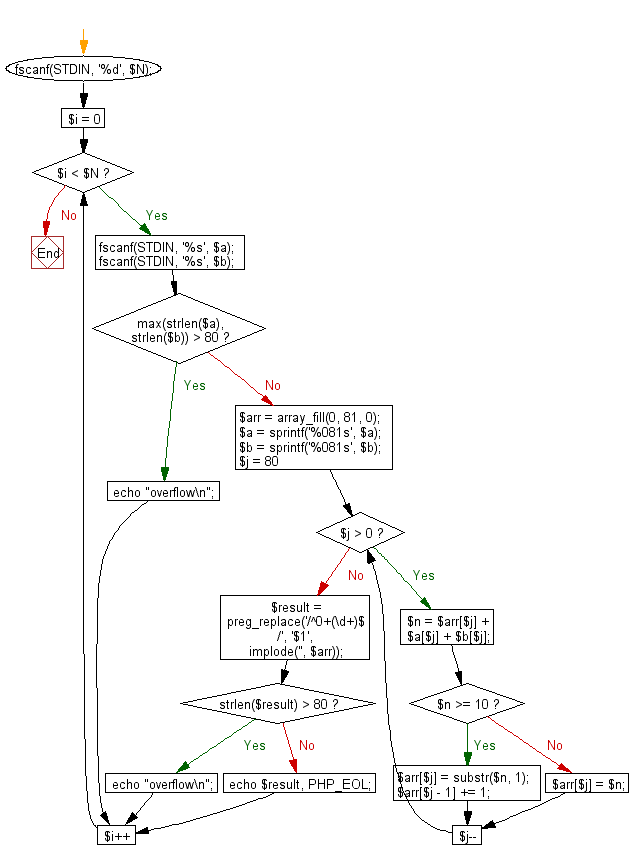﻿ PHP Exercise: Compute and print sum of two given integers - w3resource

# PHP Exercises: Compute and print sum of two given integers

## PHP: Exercise-54 with Solution

Write a PHP program to compute and print sum of two given integers (more than or equal to zero). If given integers or the sum have more than 80 digits, print "overflow".

Pictorial Presentation:Sample Solution: -

PHP Code:

``````<?php
fscanf(STDIN, '%d', \$N);
for (\$i = 0; \$i < \$N; \$i++) {
fscanf(STDIN, '%s', \$a);
fscanf(STDIN, '%s', \$b);
if (max(strlen(\$a), strlen(\$b)) > 80) {
echo "overflow\n";
continue;
}
\$arr = array_fill(0, 81, 0);
\$a = sprintf('%081s', \$a);
\$b = sprintf('%081s', \$b);
for (\$j = 80; \$j > 0; \$j--) {
\$n = \$arr[\$j] + \$a[\$j] + \$b[\$j];
if (\$n >= 10) {
\$arr[\$j] = substr(\$n, 1);
\$arr[\$j - 1] += 1;
} else {
\$arr[\$j] = \$n;
}
}
\$result = preg_replace('/^0+(\d+)\$/', '\$1', implode('', \$arr));
if (strlen(\$result) > 80) {
echo "overflow\n";
} else {
echo \$result, PHP_EOL;
}
}
?>
```
```

Sample Output:

```46
overflow
overflow
overflow
overflow
...
overflow
overflow
overflow
```

Flowchart:PHP Code Editor:

Have another way to solve this solution? Contribute your code (and comments) through Disqus.

What is the difficulty level of this exercise?

Test your Programming skills with w3resource's quiz.

﻿

## PHP: Tips of the Day

Returns all elements in an array except for the first one

Example:

```<?php
function tips_tail(\$items)
{
return count(\$items) > 1 ? array_slice(\$items, 1) : \$items;
}

print_r(tips_tail([1, 5, 7]));
?>
```

Output:

```Array
(
 => 5
 => 7
)
```